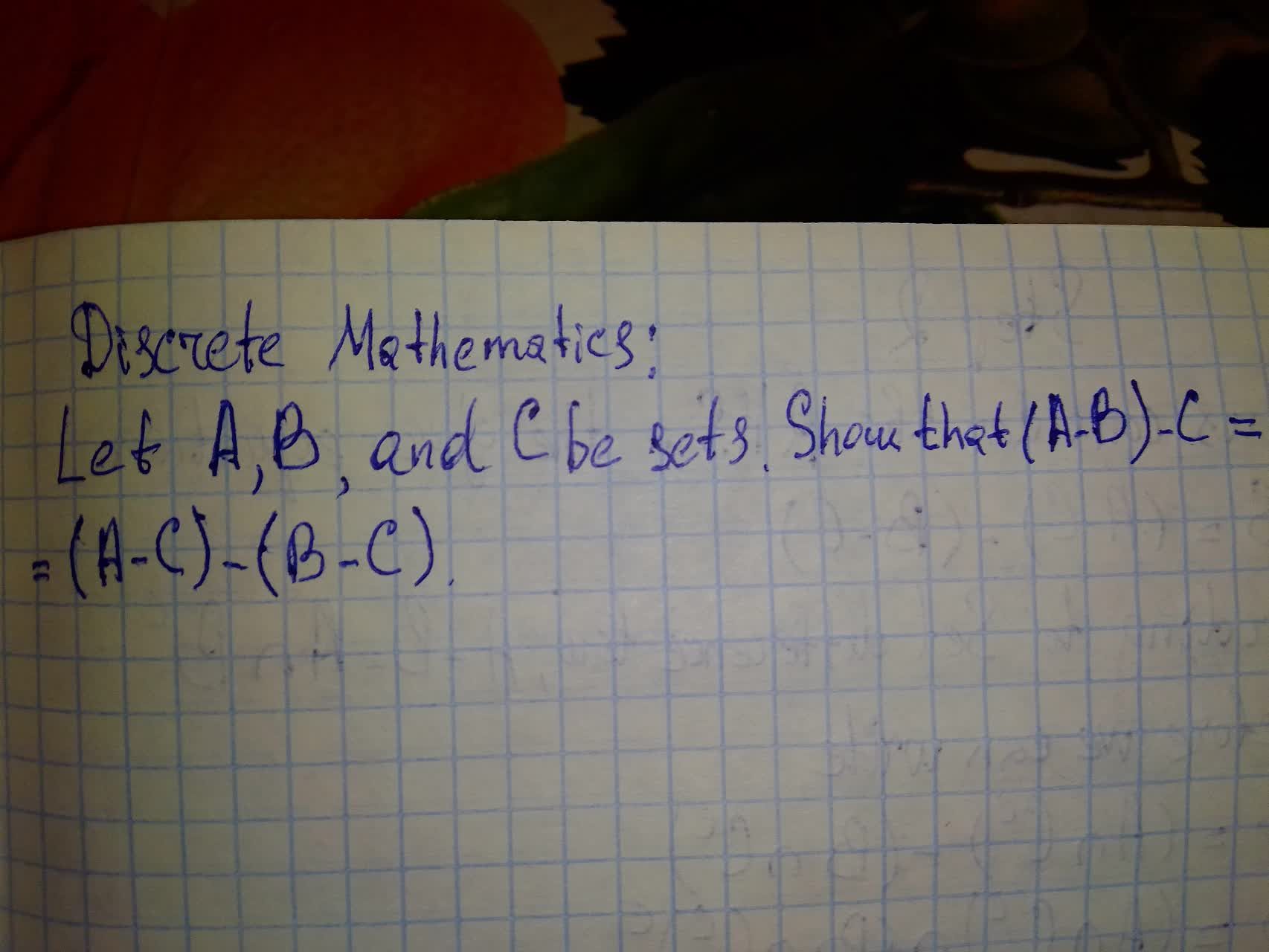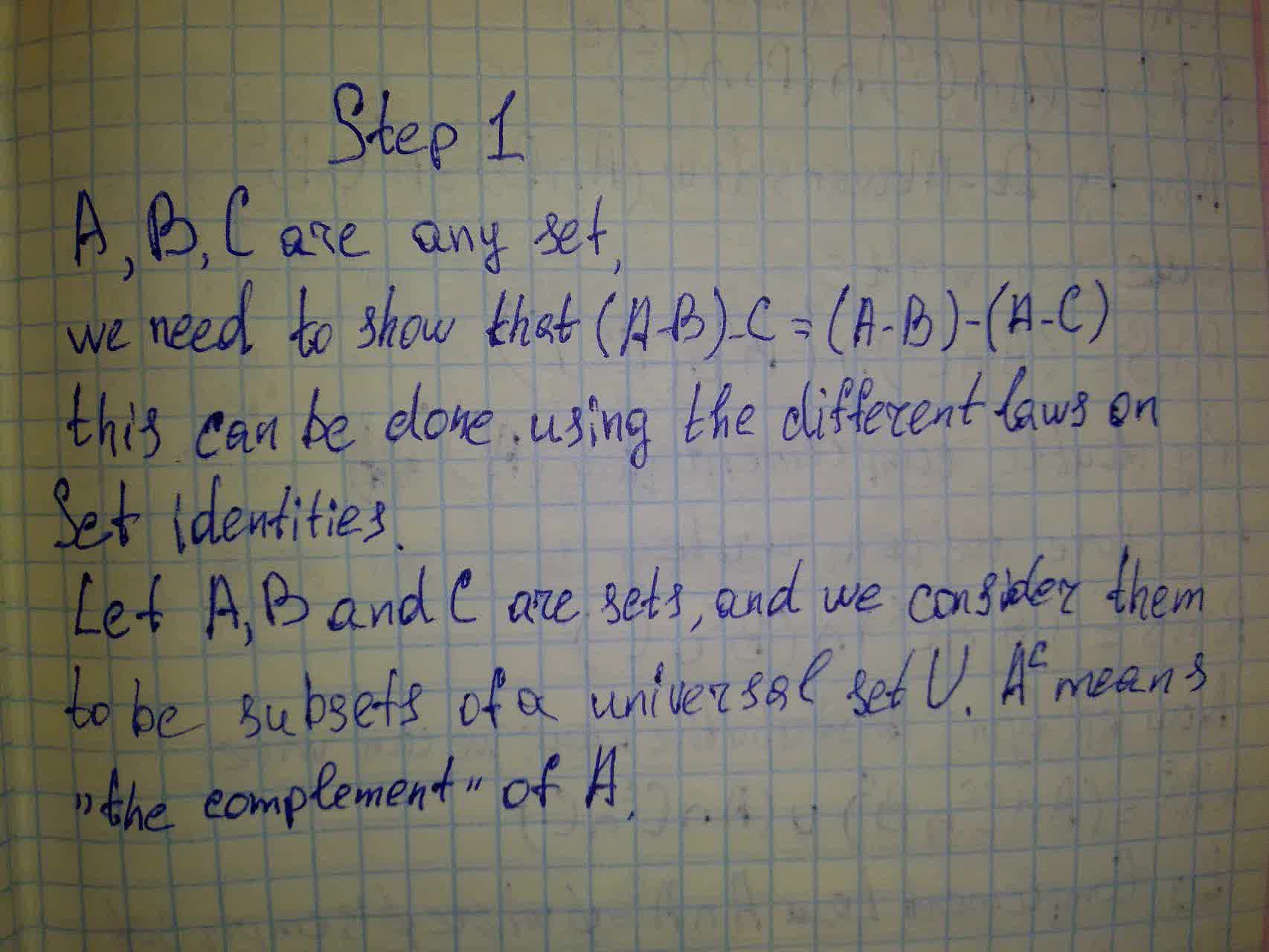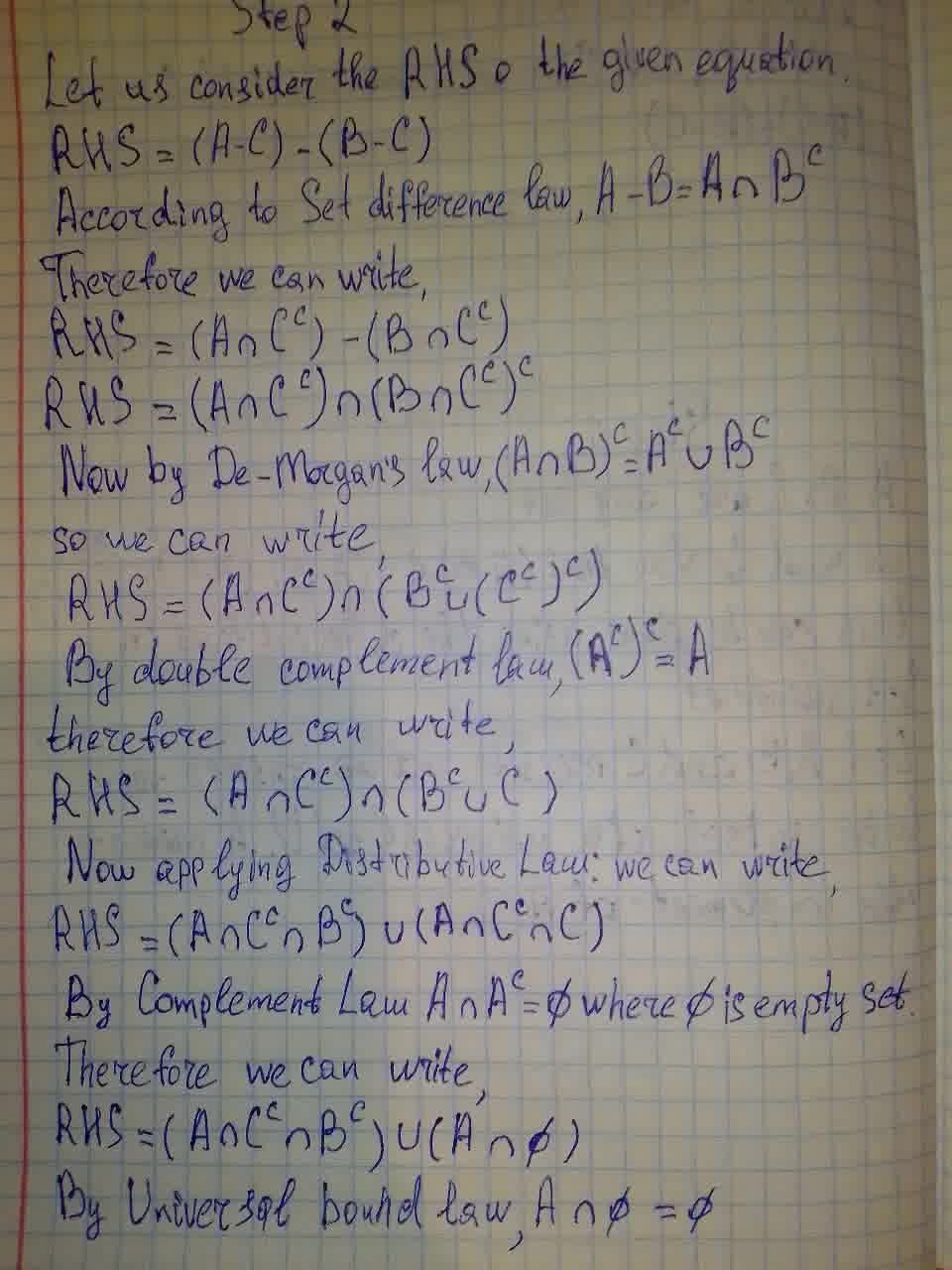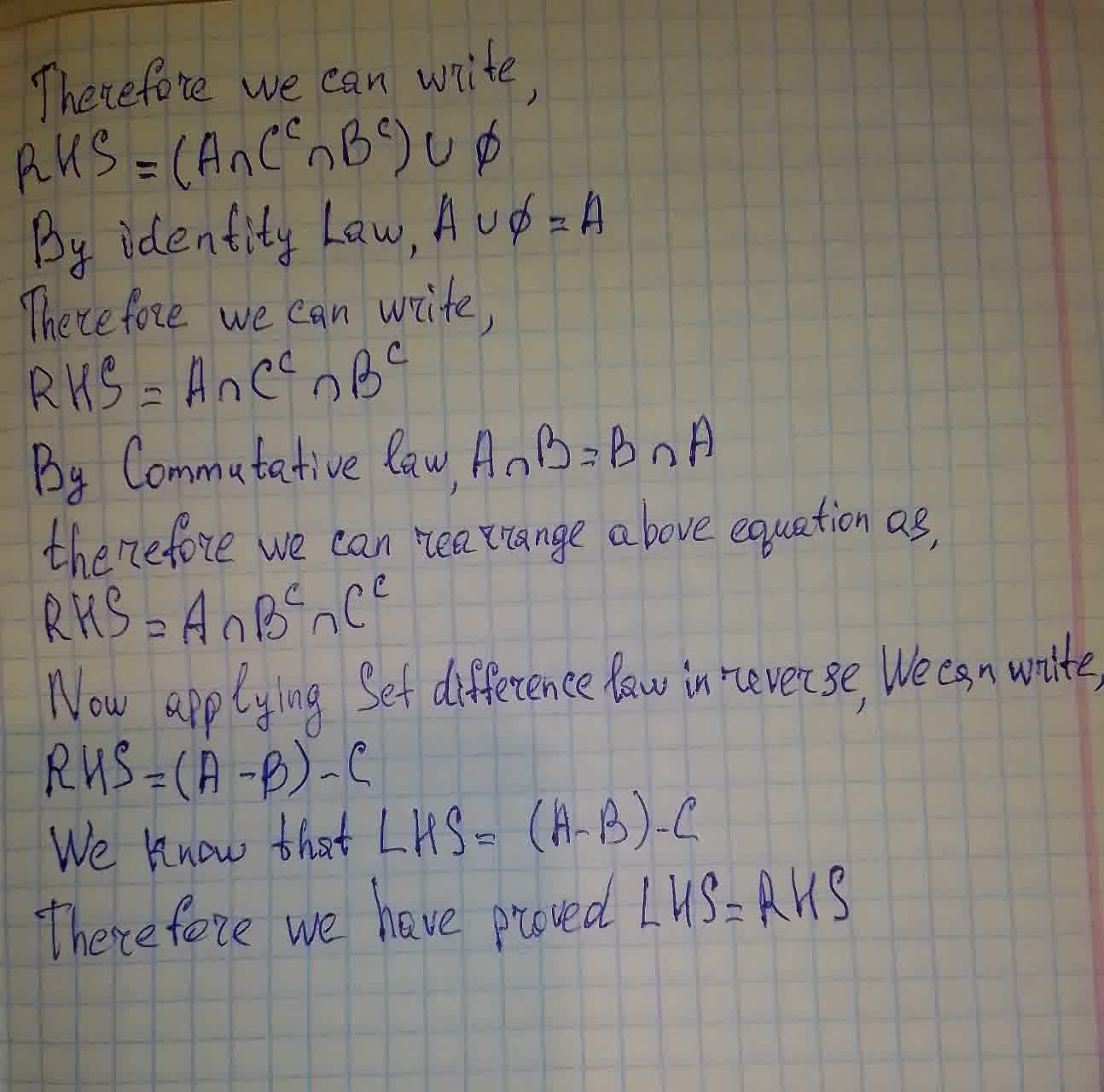Question# Let A, B, and C be sets. Show that (A-B)-C=(A-C)-(B-C)

Discrete math
ANSWEREDLet A, B, and C be sets. Show that $$\displaystyle{\left({A}-{B}\right)}-{C}={\left({A}-{C}\right)}-{\left({B}-{C}\right)}$$2021-07-29

If A, B, C is any set, then to prove the equation we need to use the various Seth identity laws